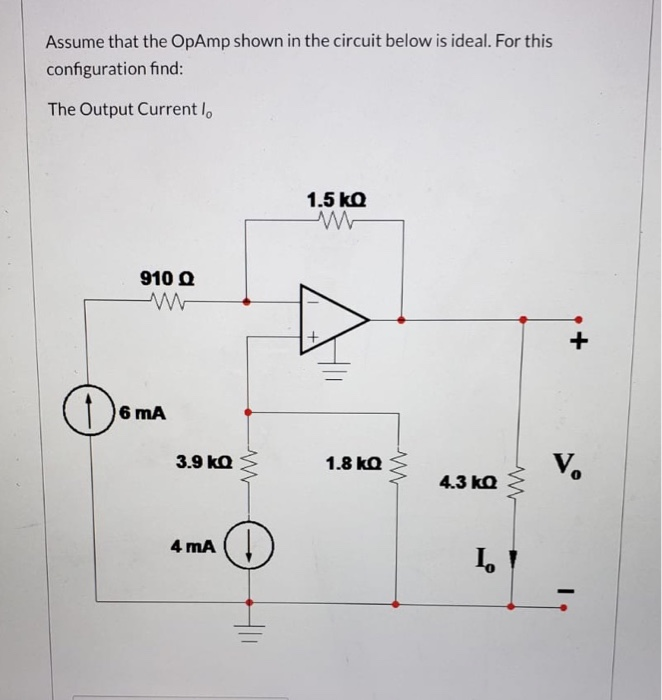# Question Assume that the OpAmp shown in the circuit below is ideal. For this configuration find: The Output Current I, 1.5 kg 9100 + o 6 mA 3.9 kg 1.8 ko V 4.3 kg 4 mA 1.GWLGAJ The Asker · Electrical EngineeringTranscribed Image Text: Assume that the OpAmp shown in the circuit below is ideal. For this configuration find: The Output Current I, 1.5 kg 9100 + o 6 mA 3.9 kg 1.8 ko V 4.3 kg 4 mA 1.
More
Transcribed Image Text: Assume that the OpAmp shown in the circuit below is ideal. For this configuration find: The Output Current I, 1.5 kg 9100 + o 6 mA 3.9 kg 1.8 ko V 4.3 kg 4 mA 1.